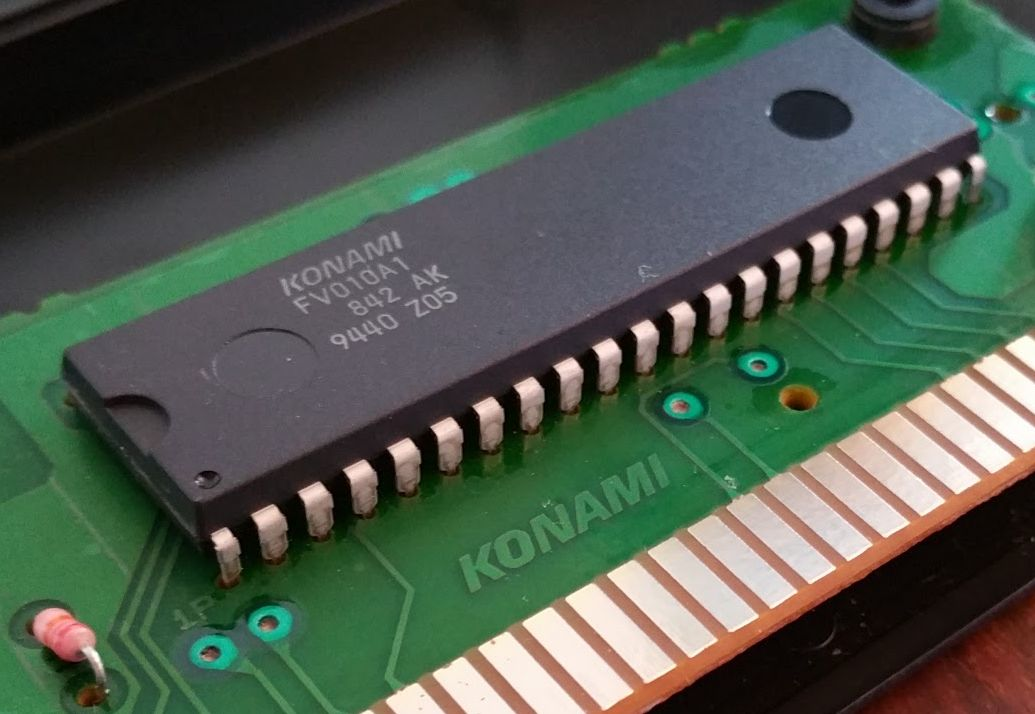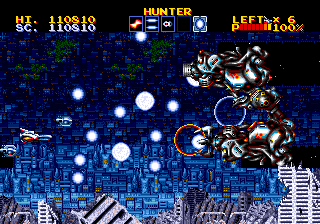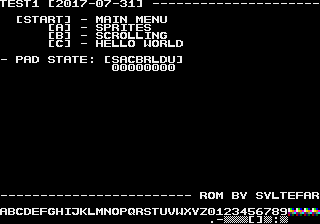# SEGA Genesis: Structure

Assembly language being lower level than other programming languages makes it very structurally open. It's an interesting language for a computer scientist to work with, as it's open for implementation of any existing or new programming paradigm.

In the following, approaches to variables and types on the Genesis will be discussed, as well as subroutine calling conventions, and an example approach to implementing the State Pattern.

## Variables

In assembly code, a variable can be represented by a register or a memory location. The Motorola 68000 has 15 normal registers `D0`-`D7` and `A0`-`A6` (`A7` is used as the stack pointer), which will work nicely for local variables in subroutines:

``````    move.w    #\$7,d0    ; int x = \$7
move.w    #\$F000,d1 ; int y = \$F000
add.w     d0,d1     ; y += x
lea       str,a0    ; char *s = str
move.b    (a0),d2   ; char c = *s
``````

Global variables are useful to represent the game state, and are most easily implemented using a fixed memory location. Here is one way to declare a variable in memory:

``````; note: my_value will be a constant on a Genesis
my_value:
ds.w    7 ; int my_value = 7
``````

The variable will be located in memory next to the code, which should work fine on architectures where the program is loaded into RAM. However, when making a SEGA Genesis ROM, the code is located in read-only memory (ROM) on the cartridge. If `my_value` is in ROM, it can never be changed and will thus be constant.Contra: Hard Corps ROM

If you want a mutable variable, you need to place it in RAM instead. A naive attempt could be to place it in address 0:

``````; note: my_value will be a constant on a Genesis
my_value = \$00000000
move.w  #7,my_value  ; my_value = 7 ?
``````

This would have worked if address 0 was in RAM on a Genesis, but it isn't. From Charles MacDonald's 'Sega Genesis hardware notes' we can get a memory map of the Genesis, where it becomes clear that address 0 is in ROM:

``````\$000000-\$3FFFFF : ROM
\$400000-\$7FFFFF : Unused (1)
\$800000-\$9FFFFF : Unused (2)
\$A00000-\$A0FFFF : Z80 address space (3)
\$A10000-\$A1001F : I/O
\$A10020-\$BFFFFF : Internal registers and expansion (4)
\$C00000-\$DFFFFF : VDP (5)
\$E00000-\$FFFFFF : RAM (6)
``````

Becase address 0 is in ROM, `my_value` in the above example is a constant. Our work RAM is in the address space `\$E00000-\$FFFFFF` (that's 2 MB). The Genesis only has 64 KB RAM (`\$10000`), but the memory is repeated over and over again in the address space. We can just limit ourselves to using `\$E00000-\$E0FFFF`.

So this works:

``````my_value = \$E00000
move.w  #7,my_value  ; my_value = 7
``````

Using the RAM address space, we can build a memory map of global variables, accessible from any part of our program:

``````mode  = \$E00000    ; 2B
xoff  = \$E00002    ; 2B
yoff  = \$E00004    ; 2B
str   = \$E00006    ; 32B string
input = \$E00026    ; 1B
``````

With this approach, avoiding variables overlapping in memory is done manually, and must be handled carefully to avoid variables overwriting each other.

In the above example, I reserved 32 B for the `str` variable, which can be interpreted as an array of 32 single characters. Arrays are discussed in further detail in the upcoming section.

## Arrays

Arrays is a key feature in most languages, and absolutely essential for game developement. MC68000 assembly code has no array concept, but arrays are easy to represent and can be accessed through constant indexes or pointers.

Let's re-use the character array `str` from the previous section:

``````str = \$E00006        ; 32 B string
; in memory: \$E00006 - \$E00025
``````

We can access any character using a constant index:

``````    lea     str,a0
move.b  0(a0),d0 ; d0 = str
move.b  1(a0),d1 ; d1 = str
``````

The addressing mode used here is called `Address register indirect with displacement`, the 'displacement' being the constant index in front of `(a0)`.

An alternative is to not use displacement, but instead update the address register `a0`, known as pointer arithmetic:

``````    lea     str,a0
move.b  (a0),d0  ; d0 = *str (str)
move.b  (a0),d0  ; d0 = *str (str)
``````

## StructuresLightening Force

The C language has the concept of a structure, a collection of variables that are closely related and next to each other in memory. The widely used concept of classes is an extension of the structure concept: a structure with an accompanying set of functions that all accept a pointer to a structure as their first argument. In high-level languages, this structure pointer (often called `this`) is usually automatically added to non-static functions as the first argument by the compiler, so programmers do not need to add it manually.

The structure concept is useful in game programming, especially when dealing with large numbers of very similar objects, such as enemies or bullets.

Any set of related variables could be considered an instance of a structure. So if we have a structure representing the position and velocity of an enemy in a game, we could have a structure like this:

``````                   ; struct enemy
enemy_x  = \$E00100 ;   int16 x
enemy_y  = \$E00102 ;   int16 y
enemy_dx = \$E00104 ;   int16 dx
enemy_dy = \$E00106 ;   int16 dy
``````

However, if we want more than one enemy at a time, it would be useful to have an array of structures. In the case of `enemy`, we could allocate a pool of `enemy` instances and update all of them every frame.

### Array of Structures: Sequential Layout

An obvious way to store an array of enemy structs in memory would be sequentially, like this:

``````Structure sequence

enemy 0    enemy 1    enemy 2         enemy N
base+\$00   base+\$08   base+\$10        base+N*8
x,y,dx,dy  x,y,dx,dy  x,y,dx,dy  ...  x,y,dx,dy
``````

For this, we need to access the structs through a pointer to the beginning of a struct in memory and offsets that represent the different member variables:

``````enemy_data  = \$E00100 ; struct enemy [N], takes up N*8 B of memory
offset_x    = 0       ;   int16 x
offset_x    = 2       ;   int16 y
offset_dx   = 4       ;   int16 dx
offset_dy   = 6       ;   int16 dy
STRUCT_SIZE = 8       ; sizeof(enemy)
``````

If we consider this simple update routine:

``````    for each enemy E
E.x += E.dx
E.y += E.dy
``````

We could implement it like this:

``````    lea     enemy_data,a0            ; i = 0; enemy e = data[i]

move.w  #N-1,d7
.loop                                ; do
move.w  offset_dx(a0),d0         ;   e.x += e.dx
move.w  offset_dy(a0),d0         ;   e.y += e.dy
add.l   #STRUCT_SIZE,a0          ;   e = data[++i]
dbra    d7,.loop                 ; while (i < COUNT)
``````

Next, we will consider an alternative approach to the sequential structure layout.

### Array of Structures: Member Arrays

As an alternative to the sequential memory layout, we could store arrays of each member of `enemy`:

``````Member array

enemy x: base+\$00
x0,x1,x2, ... xN

enemy y: base+N*2
y0,y1,y2, ... yN

enemy dx: base+(N+1)*2
dx0,dx1,dx2, ... dxN

enemy dy: base+(N+2)*2
dy0,dy1,dy2, ... dyN
``````

The update algorithm:

``````    for each enemy E
E.x += E.dx
E.y += E.dy
``````

Could be implemented using the member array approach:

``````    lea    enemy_x,a0  ; int16 *enemy_x_ptr  = enemy_x
lea    enemy_y,a1  ; int16 *enemy_y_ptr  = enemy_y
lea    enemy_dx,a2 ; int16 *enemy_dx_ptr = enemy_dx
lea    enemy_dy,a3 ; int16 *enemy_dy_ptr = enemy_dy

move.w #N-1,d0
.loop                  ; do
add.w  (a2),a0     ;   (*enemy_x_ptr) += *enemy_dx_ptr
add.w  (a3),a1     ;   (*enemy_y_ptr) += *enemy_dy_ptr
dbra    d0,.loop   ; while (i < COUNT)
``````

### Performance Analysis

The CPU of the Genesis, the Motorola 68000, does not have a cache, caching was only present in the Motorola processors 68010 and newer, so performance on the 68000 only really depends on how many clock cycles a given algorithm uses. Unlike most newer CPUs, memory access patterns are not a factor.

Let's first look at the sequential layout update routine:

``````    lea     enemy_data,a0            ; i = 0; enemy e = data[i]

move.w  #N-1,d7
.loop                                ; do
move.w  offset_dx(a0),d0         ;   e.x += e.dx
move.w  offset_dy(a0),d0         ;   e.y += e.dy
add.l   #STRUCT_SIZE,a0          ;   e = data[++i]
dbra    d7,.loop                 ; while (i < COUNT)
``````

Inside the loop, we have 3 different instructions, excluding the loop code itself:

``````    move.w  d(An),Dn ; d(An) - Address register indirect, displacement
;   Dn  - Data register direct
;               - 12 cycles

; add.w Dn,<M>  -  8 cycles
;   <M> = d(An) -  8 cycles
;                 ---------
;                 16 cycles

``````

See the Neo-Geo Development Wiki for more on MC68000 instruction timing.

Using this information, we know that the meat of the loop uses:

``````    move.w  offset_dx(a0),d0  ;        12 cycles
move.w  offset_dy(a0),d0  ;        12 cycles
; ----------------
; total: 64 cycles
``````

In total, the update algorithm takes `64 * N` cycles.

If we compare that to the member array implementation:

``````    lea    enemy_x,a0  ; int16 *enemy_x_ptr  = enemy_x
lea    enemy_y,a1  ; int16 *enemy_y_ptr  = enemy_y
lea    enemy_dx,a2 ; int16 *enemy_dx_ptr = enemy_dx
lea    enemy_dy,a3 ; int16 *enemy_dy_ptr = enemy_dy

move.w #N-1,d0
.loop                  ; do
add.w  (a2),a0     ;   (*enemy_x_ptr) += *enemy_dx_ptr
add.w  (a3),a1     ;   (*enemy_y_ptr) += *enemy_dy_ptr
dbra    d0,.loop   ; while (i < COUNT)
``````

We have 2 different instructions, excluding the loop code itself:

``````    add.w   (An),An ; Address operand calculations:
; add.w <ea>,An - 8 cycles
;   <ea> = (An) - 4 cycles
;                12 cycles
``````

Now, we can calculate the total cycle count:

``````    add.w  (a2),a0  ;        12 cycles
; ------------
; total: 56 cycles
``````

In total, the update algorithm takes `56 * N` cycles, so in this case, the member array approach is somewhat faster. It is mainly due to avoiding the use of 'Address register indirect with displacement', which is a bit slow.

## Function Calling ConventionsThe other guys just don't stack up

In many programming languages, parsing parameters into and out of functions and methods is hidden by the language implementation. For example, the formal parameters may be pushed onto the stack by the calling code before giving executing to the function body itself. In assembly code, you have to make these decisions yourself.

The simplest implementation would be defining input and output registers for a function:

``````result = Add(parameterA, parameterB)
D0             D0          D1
``````

This function could be implemented like this:

``````Add:
rts
``````

And called like this:

``````    ; D0 = Add(5,7)
move.w  #5,D0   ; parameterA = 5
move.w  #7,D1   ; parameterA = 7
``````

This works, as long as you're careful with which registers are overwritten by functions.

However, when you have multiple levels of function calls, it goes bad almost immediately:

``````Multiply:

move.w  d0,d2    ; Multiply destroys D2
.loop:
dbra    d1,.loop
rts
; result = Power( x, power )
;   D0            D0   D1

Power:
move.w  d1,d2    ; But D2 is also used by Power... Uh oh
.loop:
move.w  d2,d0
jsr     Multiply ; When this is done, D2 is destroyed
dbra    d2,.loop ; argh

rts
``````

To avoid problems, our function can save the state of the registers that will be overwritten onto the stack:

``````Multiply:

move.l  d1,-(sp)  ; push D1 onto stack
move.l  d2,-(sp)  ; push D2 onto stack

move.w  d0,d2     ; destroys D2, but we already saved it
.loop:
dbra    d1,.loop  ; here we subtract from D1

; Restore D1 and D2 from the stack.
; Since we pushed D1 before D2, we must pop them
; in the opposite order:
move.l  (sp)+,d2  ; restore D2 from the stack
move.l  (sp)+,d1  ; restore D1 from the stack

rts
``````

We can use the 'movem' instruction to save and restore multiple registers:

``````    movem.l d1-d2,-(sp)     ; push d1, d2 to the stack
movem.l (sp)+,d1-d2     ; pop d2, d1 from the stack
``````

If you don't wan't to figure out which registers are overwritten, you can save and restore all of them:

``````    movem d0-d7/a0-a6,-(sp) ;    push all registers onto the stack
movem (sp)+,d0-d7/a0-a6 ; restore all registers from the stack
``````

It's obviously faster to only push the ones you actually overwrite.

## State PatternI wanted to implement a State Pattern in 68000 assembly to make a menu structure. My first implementation is based on the way I would implement it in C#, like this:

``````delegate void StateMethod();

void Test() {
StateMethod state = StateA;
state();
}
void StateA() { }
void StateB() { }
``````

The 68000 assembly version could look like this:

``````state = \$E00000            ; function ptr location
move.l  #StateA,state   ; state = StateA
.loop;
move.l  state,a0        ; a0 = *(state)
jsr     (a0)            ; jumps to a0, call StateA
bra     .loop
StateA:
rts
StateA:
rts
``````

I found an equivalent implementation in the disassembled code of Sonic the Hedgehog, which was based on a jump table:

``````MainGameLoop:
move.b	(v_gamemode).w,d0      ; load Game Mode
andi.w	#\$1C,d0
bra.s	MainGameLoop
GameModeArray:

ptr_GM_Sega:	bra.w	GM_Sega		; Sega Screen (\$00)
ptr_GM_Title:	bra.w	GM_Title	; Title	Screen (\$04)
ptr_GM_Demo:	bra.w	GM_Level	; Demo Mode (\$08)
ptr_GM_Level:	bra.w	GM_Level	; Normal Level (\$0C)
ptr_GM_Special:	bra.w	GM_Special	; Special Stage	(\$10)
ptr_GM_Cont:	bra.w	GM_Continue	; Continue Screen (\$14)
ptr_GM_Ending:	bra.w	GM_Ending	; End of game sequence (\$18)
ptr_GM_Credits:	bra.w	GM_Credits	; Credits (\$1C)

rts
``````

Based on that, here is a jump table implementation of the state pattern:

``````    move.w  #0,d0             ; function index = 0 (StateA)
lsl.w   #2,d0             ; d0 *= sizeof( function pointer ) = 4
jsr     DoState(pc,d0.w)  ; invoke jump table

DoState:                      ; Jump table
bra.w     StateA          ; offset 0
bra.w     StateB          ; offset 4
rts                       ; this should never be reached
StateA:
rts
StateB:
rts
``````

I ended up using the jump table, as adding new functions was trivial compared to assigning new function pointers.

```
```